## 2.9Conservation and boundedness

The material derivative Eq. (2.14 ) includes theterm. However, the term can take for the form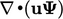, by applying conservation of mass Eq. (2.8 ), as shown in Sec. 2.8 . The two terms are related by the product rule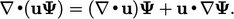(2.31)

While the two terms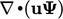and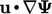appear similar, they aﬀect the solution of an equation is diﬀerent ways.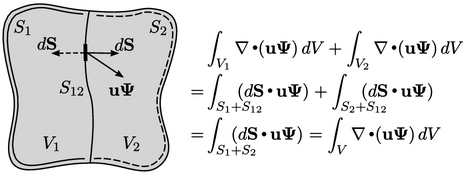Theterm maintains conservation in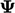. All variables are inside (to the right of) the divergence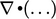, so when we integrate it over a volume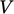, it can be entirely transformed by Gauss’s theorem to an integral of the ﬂux of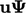at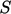.

If we splitinto two sub-volumes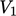and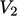, the surface integrals are also split over two surfaces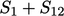and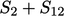, where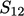is the part of the surface common to both volumes.

The ﬂuxes atfor each sub-volume have equal magnitude but opposite sign, since their respective surface area vectors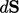point outwards from the volume in opposing directions. These ﬂuxes cancel one another out, such that the sum of integrals over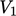and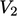is identically equal to the integral over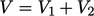.

Thus, the term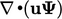ensures conservation in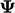across a surface separating regions of the ﬂow domain. Conservation is guaranteed at all points in the limit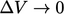for any sub-volume.

The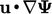form cannot transform to a surface integral so does not ensure conservation. Instead it ensures boundedness, as demonstrated by the solution ofin the following equation in one (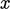) dimension (1D)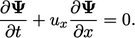(2.32)
The solution to this equation has the form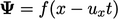. If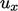is uniform, then the proﬁle of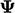does not change but simply translates at a speed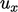as shown in the left diagram. Ifvaries with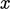, the proﬁle changes, e.g. ﬂattens, as shown in the right diagram.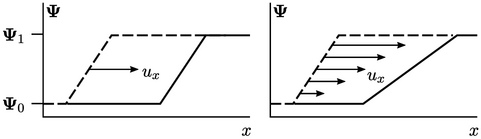In both cases, theproﬁle remains within the original bounds of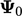and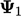; the solution is said to be bounded. While the behaviour is illustrated in 1D, it extends to 3D.

Thus, the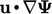term ensures boundedness. By contrast, the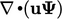term, while ensuring conservation, does not ensure boundedness when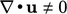. The two terms are connected by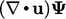which changes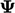due to expansion/contraction of the ﬂuid, as discussed in Sec. 2.4 . Its eﬀect is illustrated by ﬂattening of the proﬁle in the right diagram, since a non-uniform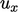corresponds to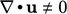.

Notes on CFD: General Principles - 2.9 Conservation and boundedness